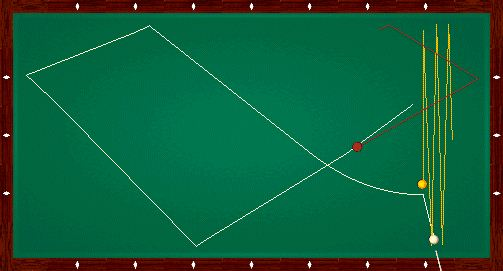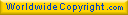En Français Home Billiards Hydraulic ram HNS Relativity Botany Music Ornitho Meteo Links/Contact Theory of the Game The Art Bibliography

# Regis Petit's Website

## Physics of Billiards1. Physics of Billiards
2. Websites
3. Another ball games

### 1. Physics of Billiards

Book "Billard - Théorie du jeu" [in English : "Billiards : Theory of the Game"]
Beyond thinking and analysis of our main champions, it is good to get back to what can be likened to a Billiards treatise...

The Art of Billiards Play (Pour la Science N°246, Avril 1998)
A knowledge of the laws of physics ensures a more reasoned and confident game. Physics both determines and explains the game's playing parameters...

Bibliography
Reference list on Billiards...

Other books
Huybrechts Guy's collection (Collection - Books - Lists - Miscellaneous)...

C'est du billard
Reportage "Archimède". Le magazine scientifique diffusé sur Arte (reproduction de l'article "http://archives.arte-tv.com/hebdo/archimed/19980331/ftext/sujet4.html" qui n'est plus disponible)...

C'est pas sorcier - Bille en tête : le billard (Youtube, 25:59)
Reportage "C'est pas sorcier". Le magazine de la science et de la découverte diffusé sur France 3...

### 3. Another ball games

#### 3.1. Bowling

Physics of bowling is similar to physics of billiards. The cloth corresponds to the bowling track, and the dry friction between ball and cloth becomes the viscous friction between ball and oiled track.
The equations of ball motion are identical to those of billards, except the force (F) and the vertical torque (K vertical) which become :
F = M g (z - fv WE)
K vertical = - (kz / R) (M g R) Ω vertical
fv = coefficient of viscous friction between ball and track (in s/m)
kz = coefficient of boring viscous friction between ball and track (in s.m)

The vector resolution gives the following results :
W = Wi - (1 - exp[ -j t ]) (2/7) WEi
Ω horizontal = Ωi horizontal - (5/ 2R) z x (W - Wi)
Ω vertical = exp[ -k t ] Ωi vertical
F = M g (z - fv exp[ -j t ] WEi)
WEi = Wi + R z x Ωi
j = (7/2) fv g
k = (5/2) (1/ R2) kz g
The ball slides on the oiled track and never reaches the rolling without slipping.
The trajectory (OG) of the center of gravity G is given by the following equation :
OG = OGi + t Wi - (t + (1/j) exp[ -j t ] - (1/j)) (2/7) WEi
Nota : This trajectory is not a parabola as billiards.
The rotation angles (A) of the ball around the G center are given by the following equations :
A horizontal = Ai horizontal + t Ωi horizontal + (5/ 7R) (1/j) (j t + exp[ -j t ] - 1) z x WEi
A vertical = Ai vertical + (1/k) (1 - exp[ -k t ])) Ωi vertical

#### 3.2. Golf or football

Physics of golf or football is more complex than physics of billiards. The cloth corresponds to the air surrounding the ball, and the dry friction between ball and cloth becomes a sum of three factors : resistance to movement (drag Tr), resistance to own rotation (resistant torque Cr) and Magnus effect due to the own rotation (lift Po).
The high velocity of ball through air gives a turbulent flow. The equations of ball motion including the wind speed become :
M dW/dt = F - M g z
I dΩ/dt = Cr
F = Tr + Po
Tr = - kr ||W - W wind|| (W - W wind)
kr = (1/2) Cx (r air) π R2
Cx = drag coefficient (0.14 for a sphere)
r air = density of air
Po = kp Ω x (W - W wind)
kp = lift coefficient
Cr = - kc Ω
kc = viscous friction coefficient

Vector resolution is complex but reachable under some simplifying conditions.

Copyright © 2005 Régis Petit.Last page update : October 30, 2021.